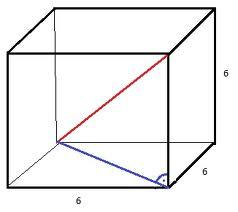# Diagonal

Determine the dimensions of the cuboid, if diagonal long 53 dm has an angle with one edge 42° and with another edge 64°.

a =  39.3867 dm
b =  23.2337 dm
c =  26.7934 dm

### Step-by-step explanation:Did you find an error or inaccuracy? Feel free to write us. Thank you!Tips to related online calculators
Do you want to convert length units?
Pythagorean theorem is the base for the right triangle calculator.

#### You need to know the following knowledge to solve this word math problem:

We encourage you to watch this tutorial video on this math problem:

## Related math problems and questions:

• CuboidFind the dimensions of cuboid a, b, c; if diagonal d=9 dm has an angle with edge α=55° and has an angle with edge b β=58°
• Diagonals of a prismThe base of the square prism is a rectangle with dimensions of 3 dm and 4 dm. The height of the prism is 1 m. Find out the angle between the body diagonal with the diagonal of the base.
• Angle of diagonalThe angle between the body diagonal of a regular quadrilateral and its base is 60°. The edge of the base has a length of 10cm. Calculate the body volume.
• Resultant forceCalculate mathematically and graphically the resultant of three forces with a common center if: F1 = 50 kN α1 = 30° F2 = 40 kN α2 = 45° F3 = 40 kN α3 = 25°
• Elevation anglesFrom the endpoints of the base 240 m long and inclined at an angle of 18° 15 ', the top of the mountain can be seen at elevation angles of 43° and 51°. How high is the mountain?
• Base diagonalIn a regular 4-sided pyramid, the side edge forms an angle of 55° with the base's diagonal. The length of the side edge is eight meters. Calculate the surface area and volume of the pyramid.
• Space diagonal anglesCalculate the angle between the body diagonal and the side edge c of the block with dimensions: a = 28cm, b = 45cm and c = 73cm. Then, find the angle between the body diagonal and the plane of the base ABCD.
• Prism diagonalThe body diagonal of a regular square prism has an angle of 60 degrees with the base, the edge length is 10 cm. What is the volume of the prism?
• CloudsFrom two points A and B on the horizontal plane was observed forehead cloud above the two points under elevation angle 73°20' and 64°40'. Points A , B are separated by 2830 m. How high is the cloud?
• Tetrahedral pyramidDetermine the surface of a regular tetrahedral pyramid when its volume is V = 120 and the angle of the sidewall with the base plane is α = 42° 30'.
• BearingA plane flew 50 km on a bearing 63°20' and the flew on a bearing 153°20' for 140km. Find the distance between the starting point and the ending point.
• TrianglesFind out whether given sizes of the angles can be interior angles of a triangle: a) 23°10',84°30',72°20' b) 90°,41°33',48°37' c) 14°51',90°,75°49' d) 58°58',59°59',60°3'What is the volume of a quadrilateral oblique prism with base edges of length a = 1m, b = 1.1m, c = 1.2m, d = 0.7m, if a side edge of length h = 3.9m has a deviation from the base of 20° 35' and the edges a, b form an angle of 50.5°.Calculate the size of the remaining sides and angles of a right triangle ABC if it is given: b = 10 cm; c = 20 cm; angle alpha = 60° and the angle beta = 30° (use the Pythagorean theorem and functions sine, cosine, tangent, cotangent)Calculate the perimeter and the area of a rectangle if its diagonal is 14 cm and the diagonals form an angle of 130°.Find the deviation of the lines AG, BH in the ABCDEFGH box-cuboid, if given | AB | = 3cm, | AD | = 2cm, | AE | = 4cmCalculate the volume and surface area of a regular quadrangular prism whose height is 28.6cm and the body diagonal forms a 50-degree angle with the base plane.